# Irrational number

(diff) ← Older revision | Latest revision (diff) | Newer revision → (diff)

A number that is not a rational number (i.e. an integer or a fraction). Geometrically, an irrational number expresses the length of a segment that is incommensurate with the segment of unit length. Already the ancient mathematicians knew of the existence of incommensurate segments. They knew, e.g., that the diagonal and side of a square are incommensurate, which is equivalent to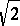being irrational.

Every real number can be written as an infinite decimal fraction, and the irrational numbers (and only they) can be written as non-periodic decimal fractions, e.g.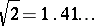,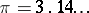. Irrational numbers determine cuts (cf. Dedekind cut) in the set of rational numbers for which there is no largest number in the lower class and no smallest number in the upper class. The set of irrational numbers is everywhere dense on the real axis: Between any two numbers there is an irrational number. The set of irrational numbers is uncountable, is a set of the second category and has type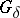(cf. Category of a set; Set of type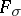()).

Irrational algebraic numbers (in contrast to transcendental numbers) do not allow for approximation of arbitrary order by rational fractions. More precisely, for any irrational algebraic numberof degreethere exists asuch that for any integersand(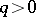) one has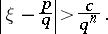Quadratic irrationalities, and only they, can be expressed by periodic continued fractions.

In fact, one can prove that for anythere is asuch that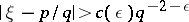if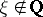is algebraic (Roth's theorem). The numbers,,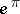are known to be irrational (even transcendental, cf. Transcendental number). However, it is not known whether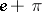,are irrational or not.